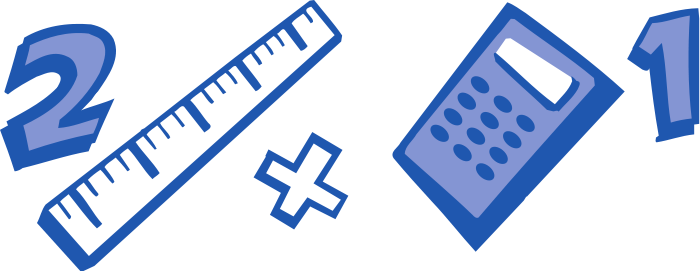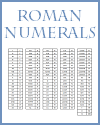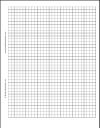﻿
Math - Free Printables, Lesson Plans, Test Prep Games | Student Handouts
 Share the learning joy!
 Math Teaching Materialswww.studenthandouts.com > Mathematics
Kindergarten

Counting and Cardinality - Know number names and the count sequence. Count to tell the number of objects. Compare numbers.

Operations and Algebraic Thinking - Understand addition, and understand subtraction.

Number and Operations in Base Ten - Work with numbers 11-19 to gain foundations for place value.

Measurement and Data - Describe and compare measurable attributes. Classify objects and count the number of objects in each category.

Geometry - Identify and describe shapes. Analyze, compare, create, and compose shapes.

Grade 1 Geometry - Reason with shapes and their attributes.

Grade 1 Measurement and Data - Measure lengths indirectly and by iterating length units. Tell and write time. Represent and interpret data.

Grade 1 Number and Operations in Base Ten - Extend the counting sequence. Understand place value. Use place value understanding and properties of operations to add and subtract.

Grade 1 Operations and Algebraic Thinking - Represent and solve problems involving addition and subtraction. Understand and apply properties of operations and the relationship between addition and subtraction. Add and subtract within 20. Work with addition and subtraction equations.

Grade 2 Geometry - Reason with shapes and their attributes.

Grade 2 - Measurement and Data - Measure and estimate lengths in standard units. Relate addition and subtraction to length. Work with time and money. Represent and interpret data.

Grade 2 Number and Operations in Base Ten - Understand place value. Use place value understanding and properties of operations to add and subtract.

Grade 2 Operations and Algebraic Thinking - Represent and solve problems involving addition and subtraction. Add and subtract within 20. Work with equal groups of objects to gain foundations for multiplication.

Grade 3 Geometry - Reason with shapes and their attributes.

Grade 3 Measurement and Data - Solve problems involving measurement and estimation. Represent and interpret data. Geometric measurement: understand concepts of area and relate area to multiplication and to addition. Geometric measurement: recognize perimeter.

Grade 3 Number and Operations - Fractions - Develop understanding of fractions as numbers.

Grade 3 Number and Operations in Base Ten - Use place value understanding and properties of operations to perform multi-digit arithmetic.

Grade 3 Operations and Algebraic Thinking - Represent and solve problems involving multiplication and division. Understand properties of multiplication and the relationship between multiplication and division. Multiply and divide within 100. Solve problems involving the four operations, and identify and explain patterns in arithmetic.

Geometry - Graph points on the coordinate plane to solve real-world and mathematical problems. Classify two-dimensional figures into categories based on their properties.

Measurement and Data - Convert like measurement units within a given measurement system. Represent and interpret data. Geometric measurement: understand concepts of volume.

Number and Operations - Fractions - Use equivalent fractions as a strategy to add and subtract fractions. Apply and extend previous understandings of multiplication and division.

Number and Operations in Base Ten - Understand the place value system. Perform operations with multi-digit whole numbers and with decimals to hundredths.

Operations and Algebraic Thinking - Write and interpret numerical expressions. Analyze patterns and relationships.Roman Numerals Conversion Chart Isometric Dots Graph Paper Quarter-inch Graph Paper